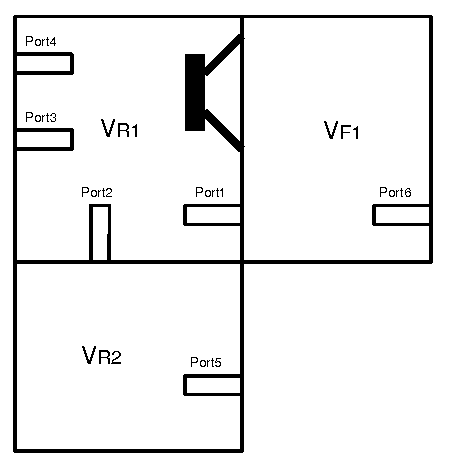# Advanced Online Speaker Box Calculator (including bandpass box)

Examples

On this page you are able to calculate an advanced speaker enclosure with Thiele/Small parameter. Diagrams are calculated for frequency response, step response, group delay, voice-coil impedance, velocity of diaphragm and of air in port, as well as displacement of diaphragm and air in ports.

## Thiele Small Parameter

Attention: You can put in your own Thiele/Small-parameter only if the speaker-select "parameterinput" is selected. To display diagrams you must fill in all appropiate fields (zeros disables the curves).

Speaker:
Resonance frequency fS (Hz):
VAS (Liter):
QTS:
QMS: => QES=0.45
DC voice-coil resistance RE (Ohm):
Voice-coil inductance LE (mH):
Effective area of the diaphragm SD (cm2): => (dD=13.16 cm)
-------------------------------------------------------
Electrical input power Pe (W): => eg=2.41 VRMS
Rg (Ohm): => QE=0.45 => QT=0.35## Enclosure Parameter:

Attention: If you chose RAP = 0, then you are calculating RAP for air in port. (look at the example diagram for acoustic resistance for port1)
Phase of Sound Pressure Frequency Response
Enclosure volume VR1 (Liter):
RAB:
RAL:
Enclosure volume VR2 (Liter):
RAB:
RAL:
Enclosure volume VF1 (Liter):
RAB:
RAL:
Port1: Vent diameter (cm): => (19.63 cm2)
Vent length (cm):
RAP:
Port2: Vent diameter (cm): => (0.00 cm2)
Vent length (cm):
RAP:
Port3: Vent diameter (cm): => (0.00 cm2)
Vent length (cm):
RAP:
Port4: Vent diameter (cm): => (0.00 cm2)
Vent length (cm):
RAP:
Port5: Vent diameter (cm): => (0.00 cm2)
Vent length (cm):
RAP:
Port6: Vent diameter (cm): => (0.00 cm2)
Vent length (cm):
RAP:
Air temperature (oC): => Cair: (343.5 m/s)

### Enclosure variant:

Calculated sound pressure etc. outside of:
VR1  (diaphragm + Port1 + Port2 + Port3 + Port4 + Enclosure losses (VR1)
VR1 + VR2  (diaphragm + Port1 + Port3 + Port4 + Port5 + Enclosure losses (VR1 + VR2)
VR1 + VR2 + VF1  (Port3 + Port4 + Port5 + Port6 + Enclosure losses (VR1 + VR2 + VF1))
VR1 + VF1  (Port2 + Port3 + Port4 + Port6 + Enclosure losses (VR1 + VF1))
(vr1=18.8, vr2=1000000000, vf1=1000000000)
Pe_max=0 Xmax=0
cas=2.49e-7 m⁵/N  mas=7.42e+1 kg/m⁴  ras=1.11e+4 Ns/m⁵  Bl=6.40e+0 N/A  rat=4.93e+4 Ns/m⁵
cms=1.35e-3 m/N  mms=1.38e-2 Kg  rms=2.06e+0 Ns/mLast Updated: 10. June 2014

Visitors: